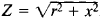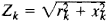# Impedance, Electrical

## Impedance, Electrical

a quantity characterizing the opposition presented by a circuit to an alternating current. Often called simply impedance, it is measured in ohms. In the case of a sinusoidal alternating current, the impedance is expressed by the ratio of the amplitude of the voltage applied to the circuit terminals and the amplitude of the current throught the circuit; here, the impedance is equal to, where r is the resistance and x is the reactance. In the case of a nonsinusoidal alternating current, the impedance is determined separately for each kth har monic component:.

Mentioned in ?
References in periodicals archive ?
However, from the viewpoints of skin-electrode impedance, electrical characteristic, and SNR value, conductive plain weave indicates a better candidate for ECG signal monitoring.
Skin-electrode impedance, electrical characteristic, signal-to-noise ratio, and comfort level are key factors to evaluate the feasibility of developed weave-based electrode in biosignals collection.

Site: Follow: Share:
Open / Close